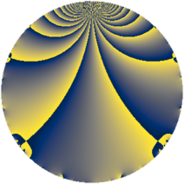# Properties

 Label 845.2.aLevel $845$ Weight $2$ Character orbit 845.a Rep. character $\chi_{845}(1,\cdot)$ Character field $\Q$ Dimension $51$ Newform subspaces $15$ Sturm bound $182$ Trace bound $3$

# Related objects

## Defining parameters

 Level: $$N$$ $$=$$ $$845 = 5 \cdot 13^{2}$$ Weight: $$k$$ $$=$$ $$2$$ Character orbit: $$[\chi]$$ $$=$$ 845.a (trivial) Character field: $$\Q$$ Newform subspaces: $$15$$ Sturm bound: $$182$$ Trace bound: $$3$$ Distinguishing $$T_p$$: $$2$$

## Dimensions

The following table gives the dimensions of various subspaces of $$M_{2}(\Gamma_0(845))$$.

Total New Old
Modular forms 104 51 53
Cusp forms 77 51 26
Eisenstein series 27 0 27

The following table gives the dimensions of the cuspidal new subspaces with specified eigenvalues for the Atkin-Lehner operators and the Fricke involution.

$$5$$$$13$$FrickeDim.
$$+$$$$+$$$$+$$$$9$$
$$+$$$$-$$$$-$$$$16$$
$$-$$$$+$$$$-$$$$16$$
$$-$$$$-$$$$+$$$$10$$
Plus space$$+$$$$19$$
Minus space$$-$$$$32$$

## Trace form

 $$51 q + 3 q^{2} + 53 q^{4} + q^{5} - 4 q^{7} + 3 q^{8} + 55 q^{9} + O(q^{10})$$ $$51 q + 3 q^{2} + 53 q^{4} + q^{5} - 4 q^{7} + 3 q^{8} + 55 q^{9} + q^{10} + 4 q^{12} + 4 q^{14} + 61 q^{16} - 2 q^{17} + 11 q^{18} + 4 q^{19} - q^{20} - 4 q^{21} - 4 q^{24} + 51 q^{25} - 12 q^{27} - 28 q^{28} + 6 q^{29} - 8 q^{30} - 12 q^{31} - q^{32} + 20 q^{33} - 6 q^{34} - 4 q^{35} + 37 q^{36} + 10 q^{37} - 48 q^{38} + 9 q^{40} + 18 q^{41} - 16 q^{42} - 12 q^{43} - 4 q^{44} + 5 q^{45} - 8 q^{46} - 12 q^{47} - 12 q^{48} + 35 q^{49} + 3 q^{50} + 20 q^{51} - 2 q^{53} + 20 q^{54} - 12 q^{55} + 4 q^{57} - 2 q^{58} - 12 q^{59} + 12 q^{60} - 6 q^{61} - 8 q^{62} + 4 q^{63} + 49 q^{64} - 68 q^{66} + 16 q^{67} - 34 q^{68} - 20 q^{69} + 16 q^{70} - 16 q^{71} - 21 q^{72} + 14 q^{73} - 46 q^{74} - 12 q^{77} - 4 q^{79} - 17 q^{80} + 67 q^{81} - 18 q^{82} + 40 q^{83} + 28 q^{84} + 6 q^{85} - 36 q^{86} - 20 q^{87} + 4 q^{88} - 2 q^{89} - 23 q^{90} - 52 q^{92} - 60 q^{93} - 20 q^{94} - 12 q^{95} - 32 q^{96} + 2 q^{97} + 51 q^{98} + 20 q^{99} + O(q^{100})$$

## Decomposition of $$S_{2}^{\mathrm{new}}(\Gamma_0(845))$$ into newform subspaces

Label Dim $A$ Field CM Traces A-L signs $q$-expansion
$a_{2}$ $a_{3}$ $a_{5}$ $a_{7}$ 5 13
845.2.a.a $1$ $6.747$ $$\Q$$ None $$1$$ $$-2$$ $$1$$ $$4$$ $-$ $+$ $$q+q^{2}-2q^{3}-q^{4}+q^{5}-2q^{6}+4q^{7}+\cdots$$
845.2.a.b $2$ $6.747$ $$\Q(\sqrt{5})$$ None $$-1$$ $$0$$ $$-2$$ $$-4$$ $+$ $+$ $$q-\beta q^{2}+(1-2\beta )q^{3}+(-1+\beta )q^{4}+\cdots$$
845.2.a.c $2$ $6.747$ $$\Q(\sqrt{13})$$ None $$-1$$ $$2$$ $$-2$$ $$-2$$ $+$ $+$ $$q-\beta q^{2}+q^{3}+(1+\beta )q^{4}-q^{5}-\beta q^{6}+\cdots$$
845.2.a.d $2$ $6.747$ $$\Q(\sqrt{3})$$ None $$0$$ $$2$$ $$2$$ $$-4$$ $-$ $+$ $$q+\beta q^{2}+(1+\beta )q^{3}+q^{4}+q^{5}+(3+\beta )q^{6}+\cdots$$
845.2.a.e $2$ $6.747$ $$\Q(\sqrt{5})$$ None $$1$$ $$0$$ $$2$$ $$4$$ $-$ $+$ $$q+\beta q^{2}+(1-2\beta )q^{3}+(-1+\beta )q^{4}+\cdots$$
845.2.a.f $2$ $6.747$ $$\Q(\sqrt{13})$$ None $$1$$ $$2$$ $$2$$ $$2$$ $-$ $+$ $$q+\beta q^{2}+q^{3}+(1+\beta )q^{4}+q^{5}+\beta q^{6}+\cdots$$
845.2.a.g $2$ $6.747$ $$\Q(\sqrt{2})$$ None $$2$$ $$0$$ $$-2$$ $$-4$$ $+$ $+$ $$q+(1+\beta )q^{2}-\beta q^{3}+(1+2\beta )q^{4}-q^{5}+\cdots$$
845.2.a.h $3$ $6.747$ $$\Q(\zeta_{14})^+$$ None $$-1$$ $$-5$$ $$3$$ $$-5$$ $-$ $-$ $$q-\beta _{1}q^{2}+(-1-\beta _{1}+\beta _{2})q^{3}+\beta _{2}q^{4}+\cdots$$
845.2.a.i $3$ $6.747$ 3.3.564.1 None $$-1$$ $$-2$$ $$3$$ $$-2$$ $-$ $-$ $$q-\beta _{1}q^{2}+(-1+\beta _{1})q^{3}+(2+\beta _{2})q^{4}+\cdots$$
845.2.a.j $3$ $6.747$ $$\Q(\zeta_{14})^+$$ None $$1$$ $$-5$$ $$-3$$ $$5$$ $+$ $+$ $$q+\beta _{1}q^{2}+(-1-\beta _{1}+\beta _{2})q^{3}+\beta _{2}q^{4}+\cdots$$
845.2.a.k $3$ $6.747$ 3.3.564.1 None $$1$$ $$-2$$ $$-3$$ $$2$$ $+$ $-$ $$q+\beta _{1}q^{2}+(-1+\beta _{1})q^{3}+(2+\beta _{2})q^{4}+\cdots$$
845.2.a.l $4$ $6.747$ 4.4.4752.1 None $$-2$$ $$-2$$ $$4$$ $$-10$$ $-$ $-$ $$q-\beta _{1}q^{2}-\beta _{3}q^{3}+(\beta _{1}+\beta _{2})q^{4}+q^{5}+\cdots$$
845.2.a.m $4$ $6.747$ 4.4.4752.1 None $$2$$ $$-2$$ $$-4$$ $$10$$ $+$ $-$ $$q+\beta _{1}q^{2}-\beta _{3}q^{3}+(\beta _{1}+\beta _{2})q^{4}-q^{5}+\cdots$$
845.2.a.n $9$ $6.747$ $$\mathbb{Q}[x]/(x^{9} - \cdots)$$ None $$-3$$ $$7$$ $$-9$$ $$-7$$ $+$ $-$ $$q+(-\beta _{1}-\beta _{6})q^{2}+(1-\beta _{4})q^{3}+(2-\beta _{3}+\cdots)q^{4}+\cdots$$
845.2.a.o $9$ $6.747$ $$\mathbb{Q}[x]/(x^{9} - \cdots)$$ None $$3$$ $$7$$ $$9$$ $$7$$ $-$ $+$ $$q+(\beta _{1}+\beta _{6})q^{2}+(1-\beta _{4})q^{3}+(2-\beta _{3}+\cdots)q^{4}+\cdots$$

## Decomposition of $$S_{2}^{\mathrm{old}}(\Gamma_0(845))$$ into lower level spaces

$$S_{2}^{\mathrm{old}}(\Gamma_0(845)) \cong$$ $$S_{2}^{\mathrm{new}}(\Gamma_0(65))$$$$^{\oplus 2}$$$$\oplus$$$$S_{2}^{\mathrm{new}}(\Gamma_0(169))$$$$^{\oplus 2}$$